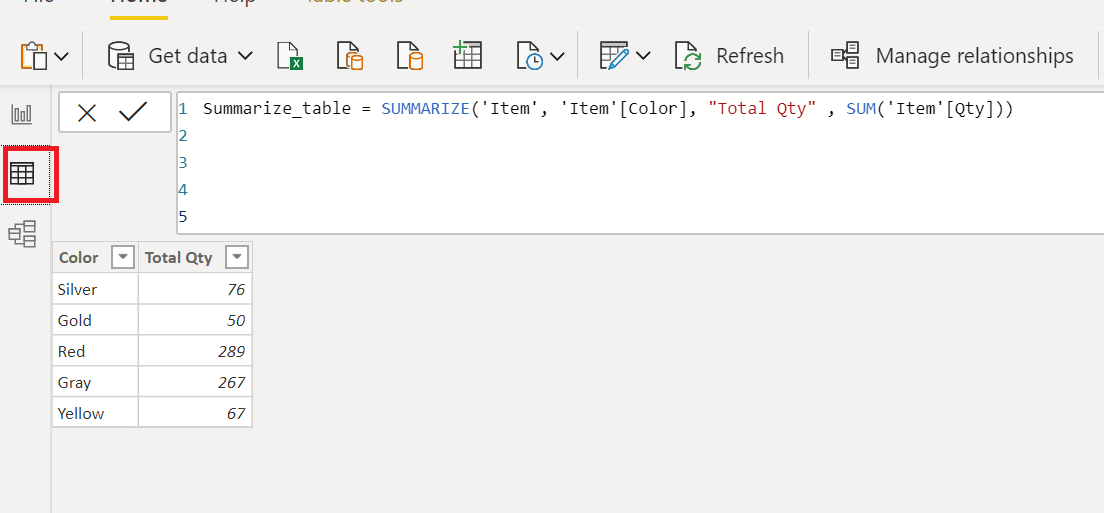Home » SUMMARIZE Function DAX

# SUMMARIZE Function DAX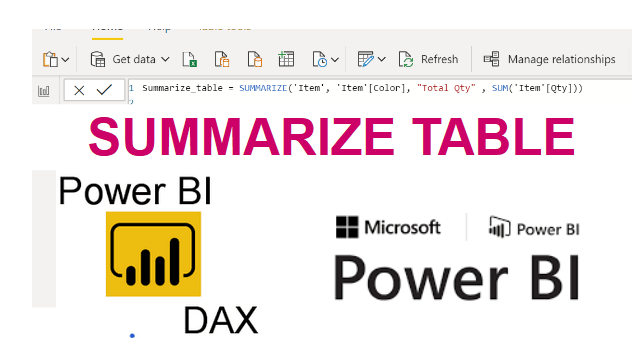### The SUMMARIZE function is a Power Bi table manipulation function in DAX that allows you to create a customized table directly in Power BI, without using Power Query.

It returns a summary table for the requested totals over a set of groups.

Syntax

```SUMMARIZE(<table>,<groupBy_columnName>[,<groupBy_columnName>]…, name>,
<expression>]…)```

<table> is a DAX expression that returns with a table of data.

<groupBy_columnName> is the qualified name of an existing column, which will be grouped based on the internal values.  This parameter cannot be an expression. It is an optional.

<name> is the name that is given to the new column that will total or summarise data. Each name must be enclosed in double quotation marks.

<expression> It may be any DAX expression that returns a single scalar value, which will be evaluated multiple times for each row, or context.

Here we have a sample dataset named as ITEM.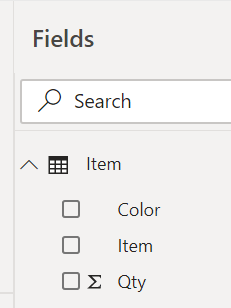You can see the data of item table as given below.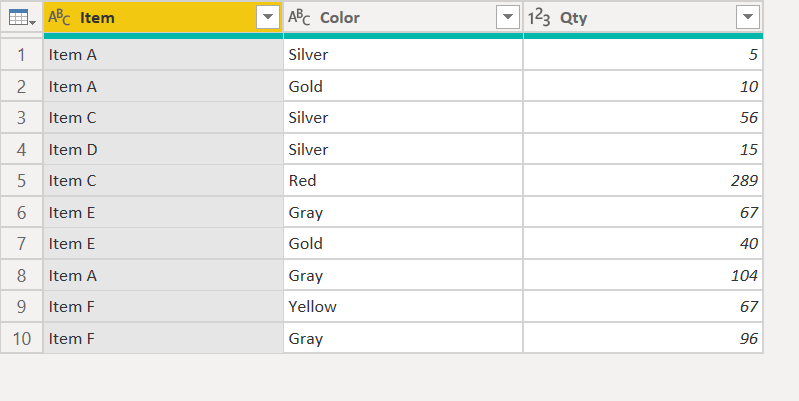Item         Color      Qty

 Item A Silver 5 Item A Gold 10 Item C Silver 56 Item D Silver 15 Item C Red 289 Item E Gray 67 Item E Gold 40 Item A Gray 104 Item F Yellow 67 Item F Gray 96

Lets summarize the Item table data based total sum of quantity by color, Baiscally Summarize table will group the rows of Item table and returns the summarise table having a total sum of quantity by color.

Lets create a SUMMARZIE table , Go to Power Bi modeling header , click on Create a new table option as given below.Now, write a following DAX that uses the summarize function Summarize_table and returns a table that holds the grouping of rows by color and total sum of quantity.

Summarize_table = SUMMARIZE(‘Item’, ‘Item'[Color], “Total Qty” , SUM(‘Item'[Qty]))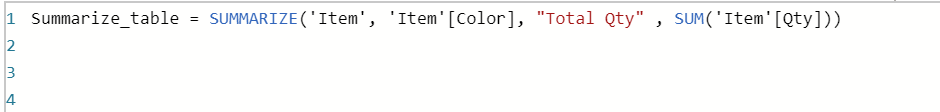Once you commit the following DAX, It creates a Summarize table in Fields pane as you can see below.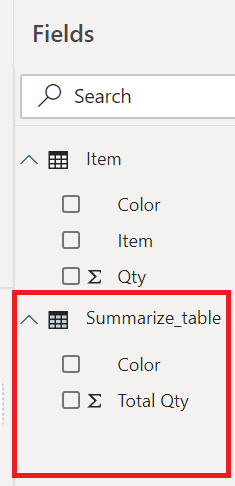Now you have a table that summaries the total quantity by color without using Power Query.

You can also validate the summarize data, Go to Data page.

Here you can see, How the summarize function groups the Item table records based on color.